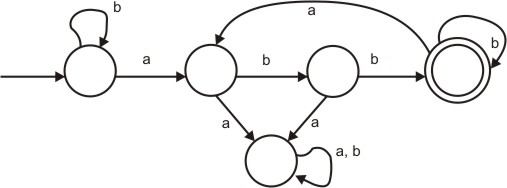# GATE | GATE-CS-2005 | Question 53

Consider the machine M:The language recognized by M is :
(A) {w ∈ {a, b}* / every a in w is followed by ex­actly two b’s}
(B) {w ∈ {a, b}* every a in w is followed by at least two b’}
(C) {w ∈ {a, b}* w contains the substring ‘abb’}
(D) {w ∈ {a, b}* w does not contain ‘aa’ as a substring}

Explanation:

Here w ∈ {a, b}* means w can be any string from the set of {a, b}* and {a, b}* is set of all strings composed of a and b (any string of a and b that you can think of) like null, a, b, aaa, abbaaa, bbbbb, aaaaa, aaaabbbbaabbababab etc.

These type of questions are frequently asked in GATE, where it is asked to choose best fit language among the options. To slove the question like this, there is a better way, we try to eliminate wrong options by choosing testing strings intelligently until we are left with one right option.As given in question, let’s we try to eliminate option (A), it recognizes only those string (composed of a and b) in which every a in w is followed by exactly two b’s , so if we take string abbb(three b’s), then it is accepted by machine , so this options is wrong. Now we try to eliminate option (C), it recognizes only those strings(composed of a and b) in which w contains the substring ‘abb’, so if we take string abbaa (has substring abb), then it is not accepted by machine, so this options is also wrong. Now we try to eliminate option (D), it recognizes only those string(composed of a and b) in which w does not contains ‘aa’ as a substring , so if we take string abbaba(‘aa’ not as a substring), then it is not accepted by machine ,so this options is also wrong. Only option with which we are left, is option (b) in which every a in w is followed by at least two b’ ,is correct.So answer is option (B).

This solution is contributed by Nirmal Bharadwaj.

My Personal Notes arrow_drop_up
Article Tags :

Be the First to upvote.

Please write to us at contribute@geeksforgeeks.org to report any issue with the above content.